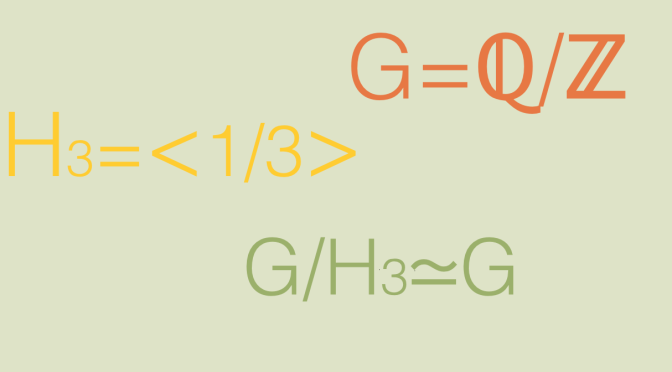# A (not finitely generated) group isomorphic to a proper quotient group

The basic question that we raise here is the following one: given a group $$G$$ and a proper subgroup $$H$$ (i.e. $$H \notin \{\{1\},G\}$$, can $$G/H$$ be isomorphic to $$G$$? A group $$G$$ is said to be hopfian (after Heinz Hopf) if it is not isomorphic with a proper quotient group.

All finite groups are hopfian as $$|G/H| = |G| \div |H|$$. Also, all simple groups are hopfian as a simple group doesn’t have proper subgroups.

So we need to turn ourselves to infinite groups to uncover non hopfian groups.

## The group $$G_1=\bigoplus\limits_{i=1}^\infty\ \mathbb Z$$

The group $$G_1$$ is an additive group and $$H_1=\mathbb Z\ \oplus\ \bigoplus\limits_{i=2}^\infty\ 0$$ is a proper subgroup. As $$G_1$$ is commutative, $$H_1$$ is normal in $$G_1$$ ($$H_1 \triangleleft G_1$$). For $$x=(x_1,x_2, \dots) \in G_1$$ we define $$\varphi(x)=(x_2,x_3, \dots) \in G_1$$. Taking $$x=(x_1,x_2, \dots),y=(y_1,y_2, \dots) \in G_1$$ we notice that:

• $$\varphi(x+y)=\varphi(x)+\varphi(y)$$,
• $$\varphi(-x)=-\varphi(x)$$,
• the value of $$\varphi$$ is constant on each class of $$G_1/H_1$$.

enabling to define a morphism $$\overline{\varphi}: G_1/H_1 \rightarrow G_1$$ which one can verify to be an isomorphism.

## The group $$G_2=(\mathbb{Q}/\mathbb{Z}, +)$$

We take an integer $$n \ge 2$$ and denote by $$a \in G_2$$ the equivalence class of $$\frac{1}{n}$$. The order of $$a$$ is equal to $$n$$. Let’s consider the function:
$\begin{array}{l|rcl} \varphi_n : & G_2 & \longrightarrow & G_2 \\ & x & \longmapsto & n \cdot x\end{array}$
As $$G_2$$ is commutative, $$\varphi_n$$ is a morphism. One can verify that $$\ker \varphi_n = H_n$$ where $$H_n$$ is the subgroup of $$G_2$$ generated by $$a$$. Moreover $$\varphi_n$$ is onto as for a representative $$p/q$$ of an element of $$\mathbb{Q}/\mathbb{Z}$$, $$p/(nq)$$ is pre-image of $$p/q$$. Finally by the first isomorphism theorem of groups, we can define an isomorphism $$\varphi_n: G_2/H_n \rightarrow G_2$$.

The groups $$G_1$$ and $$G_2$$ defined above are not finitely generated. I’ll come back later on with an example of a finitely generated group isomorphic to a proper quotient group.